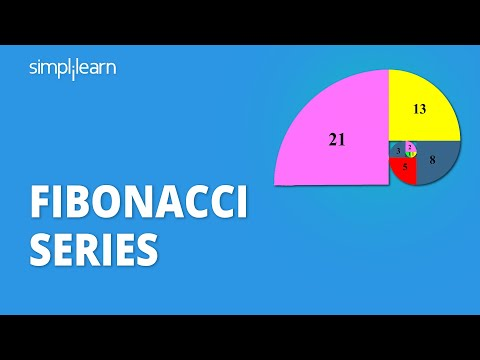### Tutorial PlaylistMathematics is more than just a set of calculations. It was created by observing patterns in the environment around us. The finest illustration of this is the Fibonacci series. It is the sequence of elements that we see in nature all the time. So, in this article, we will discover this Fibonacci Sequence in detail.

## What Is Fibonacci Series?

The Fibonacci series is a set of whole numbers in which each number is the sum of two preceding ones, starting from 0 and 1. This sequence, named after an Italian mathematician Leonardo of Pisa, AKA Fibonacci, came into the light when he introduced it to the western world in 1202.

#### Post Graduate Program: Full Stack Web Development

in Collaboration with Caltech CTMEThe Fibonacci Sequence goes like 0, 1, 1, 2, 3, 5, 8, 13, 21, and so on. While looking at this sequence, it’s difficult to guess what's so intriguing about it. So let us understand through a demonstration why it is interesting to learn about the Fibonacci series. Make different square shapes of the width of these Fibonacci numbers and place them as shown in the image below.If you join all of these boxes, you will get a subtle spiral shape, as illustrated in the image below:This shape is called a Fibonacci Spiral, responsible for developing aesthetic terms such as phi or golden ratio. You can find this shape in any natural phenomenon such as flowers, galaxies, falling water, waves, storms, etc.

For example, consider this pattern of Fibonacci spiral in Black Hole.Moving Forward, we will look into the mathematical formulation of the Fibonacci Sequence.

## Mathematical Interpretation of Fibonacci Sequence

As mentioned earlier, the Fibonacci Sequence is a set of numbers in which each number is the sum of the previous two numbers. Now, if we construct a mathematical function using the statement above, you can observe a recurrence relation:

Fib(n) =Fib(n-1) + Fib(n-2)

Let’s understand what this recurrence relation implies with the help of tabular representation for the Fibonacci series:#### New Course: Full Stack Development for Beginners

Learn Git Command, Angular, NodeJS, Maven & More## Implementation of Fibonacci Sequence Using the C Programming Language

The recurrence relation in mathematics is achieved using a programming paradigm known as recursion. It is a process that occurs when a function calls a copy of itself to work on a smaller problem. The term smaller problem indicates the reduced input size and computational complexity.

Let’s try to understand recursion with the help of an example. Consider the calculation for Fib(5). In order to calculate Fib(5), we will first have to calculate Fib(4) and Fib(3). And for these subproblems, we will again have to perform another set of computations. All the computations required to obtain Fib(5) are represented in the image below:Let’s look at the C program based on the same recurrence strategy. We are going to use a for loop in this program to print all the Fibonacci numbers computed by Fib() function in a given range.

 #include int Fib(int num) {    if ( num == 0 )       return 0;    else if ( num == 1 )       return 1;    else       return ( Fib(num-1) + Fib(num - 2) ); }   int main() {    int num;    printf("How many fibonacci numbers do you want to display on screen? ");    scanf("%d",&num);    printf("\nFibonacci numbers in sequence are: ");    int i = 0;    for ( int c = 1 ; c <= num ; c++ )    {       printf("%d ", Fib(i));       i++;    }    return 0; }

### Output:

The output console for a Fibonacci program provided user input (num) = 5.Advance your career as a MEAN stack developer with the Full Stack Web Developer - MEAN Stack Master's Program. Enroll now!

## Conclusion

In this Fibonacci Series article, you learned about the Fibonacci series and its mathematical interpretation. You also discovered some interesting facts about the Fibonacci sequence, like its presence in nature.  And finally, we discussed how recursion is used to implement the Fibonacci sequence. If you are looking for a quick and easy way to learn the Fibonacci, we advise you to watch this video: https://youtu.be/2oBgg_G3Bpk.

Every day, new products, tools, and apps are introduced into the market. Also, there are hundreds of programming languages and frameworks being utilized in the realm of software development every single day. Hence, it's crucial for you to go beyond data structure concepts and cover the foundations of interactive application development. Simplilearn's Post Graduate Program in Full Stack Web Development can prove the right solution for you to master the art of software development. Offered in collaboration with the world-renowned Caltech CTME, this bootcamp program can give you the skill and help you improve your odds of becoming a software developer fast. So, go ahead and start learning today!

If you have any questions or need clarification on any section of this article on the Fibonacci series, please leave them in the comments section at the bottom of this page; we will respond to them soon.Omkar Prabhakar Ghadage